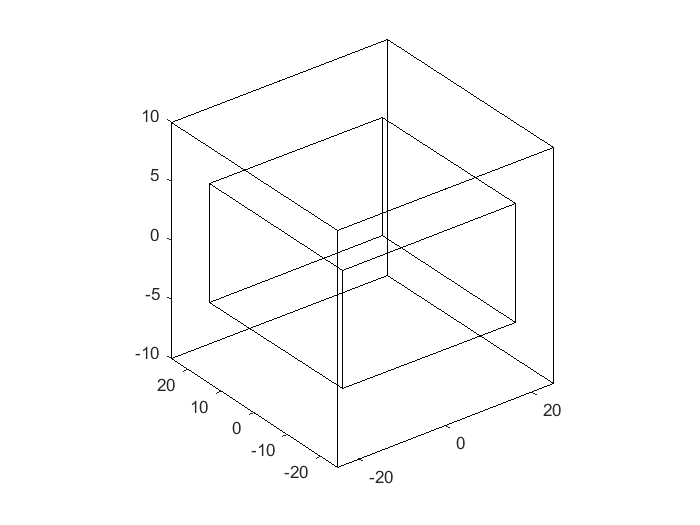• 2021-04-20 02:35:24

如何使用matlab绘制简单的正方形连续目标(离散点)

%利用linspace绘制对应长宽

%x_dim_parameter参数为取值范围

%points_num为取值点数

%为绘制成连续目标需利用meshgrid函数将正方形填满

%此时形成的是二维矩阵，需要利用reshape转为一维，

%x_dimension对应点云的x坐标，其大小为points_num * points_num2 * 2

%y_dimension对应点云的y坐标，其大小为points_num * points_num2 * 2

%z_dimension对应点云的y坐标，其大小为points_num * points_num2 * 2

%Ptar(:,4)对应点云的强度值，此处均设为1，

%Ptar为最终点云，其中Ptar(:,1)为x_dimension，其中Ptar(:,2)为y_dimension

%其中Ptar(:,3)为z_dimension，Ptar(:,4)对应点云的强度值。

x_dim_parameter2 = 0.005;

x_dim_parameter1 = -0.005;

points_num = 10;

x_dimension_mid_1 = linspace(x_dim_parameter1,x_dim_parameter2,points_num);

y_dimension_mid_1 = linspace(x_dim_parameter1,x_dim_parameter2,points_num);

[x_dimension_1,y_dimension_1] = meshgrid(x_dimension_mid_1,y_dimension_mid_1);

z_dimension_1 = 0.5*ones(points_num);

intensity_1 = ones(points_num);

x_dim2_parameter_2 = 0.025;

x_dim2_parameter_1 = -0.025;

points_num2 = 10;

x_dimension_mid_2 = linspace(x_dim2_parameter_1,x_dim2_parameter_2,points_num2);

y_dimension_mid_2 = linspace(x_dim2_parameter_1,x_dim2_parameter_2,points_num2);

[x_dimension_2,y_dimension_2] = meshgrid(x_dimension_mid_2,y_dimension_mid_2);

z_dimension_2 = 0.5*ones(points_num2);

intensity_2 = ones(points_num2);

x_dimension = cat(2,x_dimension_1,x_dimension_2);

z_dimension = cat(2,z_dimension_1,z_dimension_2);

y_dimension = cat(2,y_dimension_1,y_dimension_2);

intensity = cat(2,intensity_1,intensity_2);

Ptar(:,1) = reshape(x_dimension,[],1);

Ptar(:,2) = reshape(y_dimension,[],1);

Ptar(:,3) = reshape(z_dimension,[],1);

Ptar(:,4) = reshape(intensity,[],1);

更多相关内容
• %出内正方体框（检验质量块）； %顶点矩阵; vertex_matrix=[-0.5 -0.5 -0.5;0.5 -0.5 -0.5;0.5 0.5 -0.5;-0.5 0.5 -0.5; -0.5 -0.5 0.5;0.5 -0.5 0.5;0.5 0.5 0.5;-0.5 0.5 0.5]; %连接矩阵：连接关系矩阵每一行...
x_inner=40;     %length
y_inner=40;     %width
z_inner=10;     %height

distance_x=5;
distance_y=5;
distance_z=5;

%定义各平面与轴的交点位置；
x_outer=x_inner+2*distance_x;
y_outer=y_inner+2*distance_y;
z_outer=z_inner+2*distance_z;

x_inner1=x_inner/2;
x_inner2=-x_inner/2;
y_inner1=y_inner/2;
y_inner2=-y_inner/2;
z_inner1=z_inner/2;
z_inner2=-z_inner/2;

x_outer1=x_outer/2;
x_outer2=-x_outer/2;
y_outer1=y_outer/2;
y_outer2=-y_outer/2;
z_outer1=z_outer/2;
z_outer2=-z_outer/2;

%画出内正方体框（检验质量块）；
%顶点矩阵;
vertex_matrix=[x_inner2 y_inner2 z_inner2;x_inner1 y_inner2 z_inner2;x_inner1 y_inner1 z_inner2;x_inner2 y_inner1 z_inner2;
x_inner2 y_inner2 z_inner1;x_inner1 y_inner2 z_inner1;x_inner1 y_inner1 z_inner1;x_inner2 y_inner1 z_inner1];

%连接矩阵：连接关系矩阵每一行中的数值分别表示顶点矩阵的行标;
face_matrix=[1 2 6 5;2 3 7 6;3 4 8 7;
4 1 5 8;1 2 3 4;5 6 7 8];
patch('Vertices',vertex_matrix,'Faces',face_matrix,'FaceVertexCData',hsv(8),'FaceColor','none')

%画出外正方体框（电极笼）；
%顶点矩阵;
vertex_matrix=[x_outer2 y_outer2 z_outer2;x_outer1 y_outer2 z_outer2;x_outer1 y_outer1 z_outer2;x_outer2 y_outer1 z_outer2;
x_outer2 y_outer2 z_outer1;x_outer1 y_outer2 z_outer1;x_outer1 y_outer1 z_outer1;x_outer2 y_outer1 z_outer1];

%连接矩阵：连接关系矩阵每一行中的数值分别表示顶点矩阵的行标;
face_matrix=[1 2 6 5;2 3 7 6;3 4 8 7;
4 1 5 8;1 2 3 4;5 6 7 8];
patch('Vertices',vertex_matrix,'Faces',face_matrix,'FaceVertexCData',hsv(8),'FaceColor','none')

view(3);

axis square展开全文• ## MATLAB画正方体

千次阅读 2020-08-31 22:22:42
MATLAB画正方体 X=zeros(8,3); X([5:8,11,12,15,16,18,20,22,24])=1; d=[1 2 4 3 1 5 6 8 7 5 6 2 4 8 7 3]; plot3(X(d,1),X(d,2),X(d,3)); view(3); rotate3d;

MATLAB画正方体

X=zeros(8,3);
X([5:8,11,12,15,16,18,20,22,24])=1;
d=[1 2 4 3 1 5 6 8 7 5 6 2 4 8 7 3];
plot3(X(d,1),X(d,2),X(d,3));
view(3); rotate3d;

展开全文• 给定一个中心和一个半径，这个程序生成一个正方形的坐标，无论是空心还是实心。 该程序对于在圆形周围绘制正方形特别有用。 结果是一组适合用于在矩阵上生成正方形的坐标。
• ## MATLAB画矩形和圆

千次阅读 2020-09-28 15:20:49
以前的博文中已经讲解过如何立方体以及画圆的方法。这里将如何矩形和圆的方法写成函数形式，直接提供出来，方便大家使用。 矩形代码 % Example % cx = 1; % cy = 1; % radius = 1; % alpha = 0.8; % color = ...

## 简介

以前的博文中已经讲解过如何画立方体以及画圆的方法。这里将如何画矩形和圆的方法写成函数形式，直接提供出来，方便大家使用。

## 画矩形代码

% Example
% cx = 1;
% cy = 1;
% alpha = 0.8;
% face_color = rand(1,3);
% edge_color = rand(1,3);
% rectangle_patch(cx, cy, radius, face_color, edge_color, alpha)

function rectangle_patch(x, y, r, face_color, edge_color, alpha)
xs = [x-r, x-r, x+r, x+r];
ys = [y+r, y-r, y-r, y+r];
patch(xs, ys, face_color, 'facealpha', alpha, 'edgecolor', edge_color);
end



## 画圆代码

% Example
% cx = 1;
% cy = 1;
% alpha = 0.8;
% face_color = rand(1,3);
% edge_color = rand(1,3);
% circle_patch(cx, cy, radius, face_color, edge_color, alpha)

function circle_patch(cx, cy, radius, face_color, edge_color, alpha)
t = 0:.1:2*pi;
x = radius * cos(t) + cx;
y = radius * sin(t) + cy;
patch(x, y, face_color, 'facealpha', alpha, 'edgecolor', edge_color);
end



## 效果展开全文MATLAB画圆
• plot([1,1,0,0,1]',[0 1 1 0 0]')怎样用MATLAB画正方形，急求！！！条件没看太明白，“通过点的斜率什么意思？画正方形可以使用rectangle函数，指定左下角的顶标和边长即可。示例：% 正方形一个顶点坐标Vertex = [2 ...
• ## matlab：画正方体

千次阅读 2020-03-13 00:29:38
%Cube clear ,clc; figure; hold on; view(3); %Create four vertices V = [0 0 0;0 1 0;1 1 0;1 0 0; 0 0 1;0 1 1;1 1 1;1 0 1]; F = { [1 2 3 4] [5 6 7 8] [1 2 6 ...
• ^ 抱歉之前赶着吃饭没看清题目 clc clear all close all x1=0:0.1:2;...%轨迹如果不需要删掉即可 for i = 1: length(traj_x) hold on plot(x(i,:),y(i,:)); axis([-11,15,-0.5,110]); pause(0.1); end
• 给图像添加网格：grid on 调整坐标轴：axis axis square——>正方形图像 axis equal——>间距相同 axis auto ——> 自动选择 线条风格 z 实线 '-' z 虚线 '--' z 虚点线 '-.' z 点线 ':' 颜色说明符 白色 w ...开发语言
• 在此之前先介绍一个矩形的函数：rectangle('Position',[0.59,0.35,3.75,1.37],'Curvature',[0,0],'LineWidth',2,'LineStyle','--')rectangle('Position',[x坐标,y坐标,宽,高],'Curvature',[曲率纵向,曲率横向],'...
• %本程序出在正方体内嵌套1/4圆的组合图形 %程序虽小，但是蕴含着用结构体设置图形属性和图形对象及坐标轴属性底层设置 clc; x=0:0.01:1; %x的范围标定 y=sqrt(1-x.^2); %函数y的表达式 figure; h=plot...
• 此代码仅用于测试 OWI ROBOT ARM 在添加编码器并使用电机驱动器通过 PWM 改变电机速度时是否确实可以绘制正方形
• 当自己做出图后，发现只有将作者原图截图下来，放在某些软件中(如excel,origin)再添加上自己的图，并且要不断的调整取值范围等。图片较少，也就罢了；图片很多，或者多次算出结果，再去调整这些细节，很费时。本人...
• 任意正六边形随机撒点是通信仿真里经常用到的，无奈网上资料很少，参照...废话不多说了，先来看看怎么正六边形。theta =linspace(0,2*pi,7);plot(cos(theta),sin(theta),'g-');两句代码就可以出一个正六边形了，...
• ## matlab画三角形，多边形

万次阅读 多人点赞 2015-03-03 19:57:04
没有找到直接多边形的函数，只能是将各个点的坐标保存在数组中，将一个点与其相邻的点相连，并将最后一个点与第一个点连接。   clear; clc; a =[ 0 2 4 6 8 10 12 14 ; 0 2 1 4 6 ...
• ## matlab画温度分布图

千次阅读 2021-04-18 12:09:17
传热学MATLAB温度分布大作业完整版_工学_高等教育_教育专区。东南大学能源与环境学院 课程作业报告作业名称:传热学大作业——利用 matlab 程序解决热传导问题 院系:......三维火柴杆图。 >> x=[1:0.5:20]; >...
• 今天在用MATLAB编程的时候，用到了已知圆心和半径，画圆的程序，上网搜了一下，主要有下面两种，在这里总结一下：(这里我都是放在函数中做的，想多个圆的话可以加个for循环调用一下函数，或者直接用向量做都是可以...
• 这个简单的函数使用 Matlab 的 Mapping Toolbox 生成具有矩形边界的北极和南极的极地立体图。 通常，Matlab 中的极地立体图以圆形边界显示，但这通常不是显示有关南大洋或北极系统信息的最节省空间的方式。 Ncpolarm...
• 标记形式有'+'，'o'，'*'，和'x'，填入's'代表正方形，'d'代表菱形，'A'为上三角形，'v'为下三角形，’>’为右三角形，'<’为左三角形，'p’为五角星形，’h'为六角星形，none为不用标记。 ...
• 线型 说明 y 黄色 m 品红色 c 青蓝色 r 红色 g 绿色 b 蓝色 w 白色 k 黑色 相关属性 MATLAB图形允许控制下列直观特征： LineWidth - 指定线条的宽度（以磅为单位）。 MarkerEdgeColor - 指定标记颜色或填充标记...
• 在turtlebot端：[turtlebot] 启动Turtlebotroslaunch turtlebot_bringup minimal.launch[turtlebot] 启动雷达roslaunch rplidar_ros rplidar.launch在Matlab端：运行avoiding_obstacles.m文件运...
• ## matlab怎么画圆柱

千次阅读 2021-04-18 02:57:21
Matlab 函数图像一、螺旋线 1.静态螺旋线 a=0:0.1:20*pi...例 在区间[0,2π]sin(x),并分别标注“sin(x)””cos(x)”. 解 x=linspace(0,2*pi,30); y=sin(x); MATLAB liti3 z=cos(x); plot(x,y,x,......最终效果...
• 请问怎样用matlab画一个透明的球体,球体圆心为(x,y,z)坐标的原点[x,y,z]=sphere(30);%30是画出来的球面的经纬分面数...30的话就是30个经度,30个纬度x=4+7*x;&matlab 如何画三维空间中的离散点颜色举个例子：z=0:...
• ## matlab画三角形网格

千次阅读 2021-04-18 05:25:44
而应力解的 结束......matlab画网格并填充用于相关系数图示_数学_自然科学_专业资料。画网格并填充...对于平面问题,结构离散常用的网格形状有三角形、矩形...本章将详细介绍如何利用 Matlab 语言 编制三角形常...(前...
• ## matlab画实心圆点

千次阅读 2021-04-28 08:14:13
hist 累计图 rose 极座标累计图 stairs 阶梯图 stem 针状图 fill 实心图 feather 羽毛图 compass 罗盘图 quiver 向量场图 Matlab 如何出一个二维数组的曲线? ...一、Matlab 绘图中用到的直线属性包括: (1)...
• ## Matlab计算轮廓内切圆

千次阅读 多人点赞 2019-06-22 20:21:32
当然由于全是个人的理解肯定有不妥当的地方，权作抛砖引玉），我最终用Matlab（2018a）造了个自认为效果还可以的程序，于是有了这篇文章（偷懒的我直接从参考网站上截取了图片，权作问题的直观描述）。 在设计最终的...多边形
• ## matlab画极坐标

千次阅读 2021-04-20 02:42:12
4.3 其它图形函数除plot等基本绘图命令外,Matlab系统提供了 许多其它特殊绘图函数,这里举一些代表性 例子......三维曲线的绘制 3.3 三维网线图和曲面图的绘制 3.4 Matlab图形处理的基本技术 3.5 动画技术 3.6 图形...
• 请问各位用MATLAB画出的图形(分形天线，编程画出来的)，如何导入到仿真软件中(如HFSS)呢?保存的时候要选择哪种格式啊?同问。没作过，不知道行不行。好像是不行。同问。先行谢谢咯，那位哥么能帮忙解决一下啊……无法...
• MATLAB 中提供了一系列的函数用于绘制不同形式... contour(z):......(x,y,z) 有等位线投影的实心网格图 [x,y,z]=cylinder(r,n) 三维旋转曲面,其中n为母线的条数 Matlab软件例1:在区间[0,10π]出参数曲线x=sint, y=......

# matlab画正方形matlab 订阅